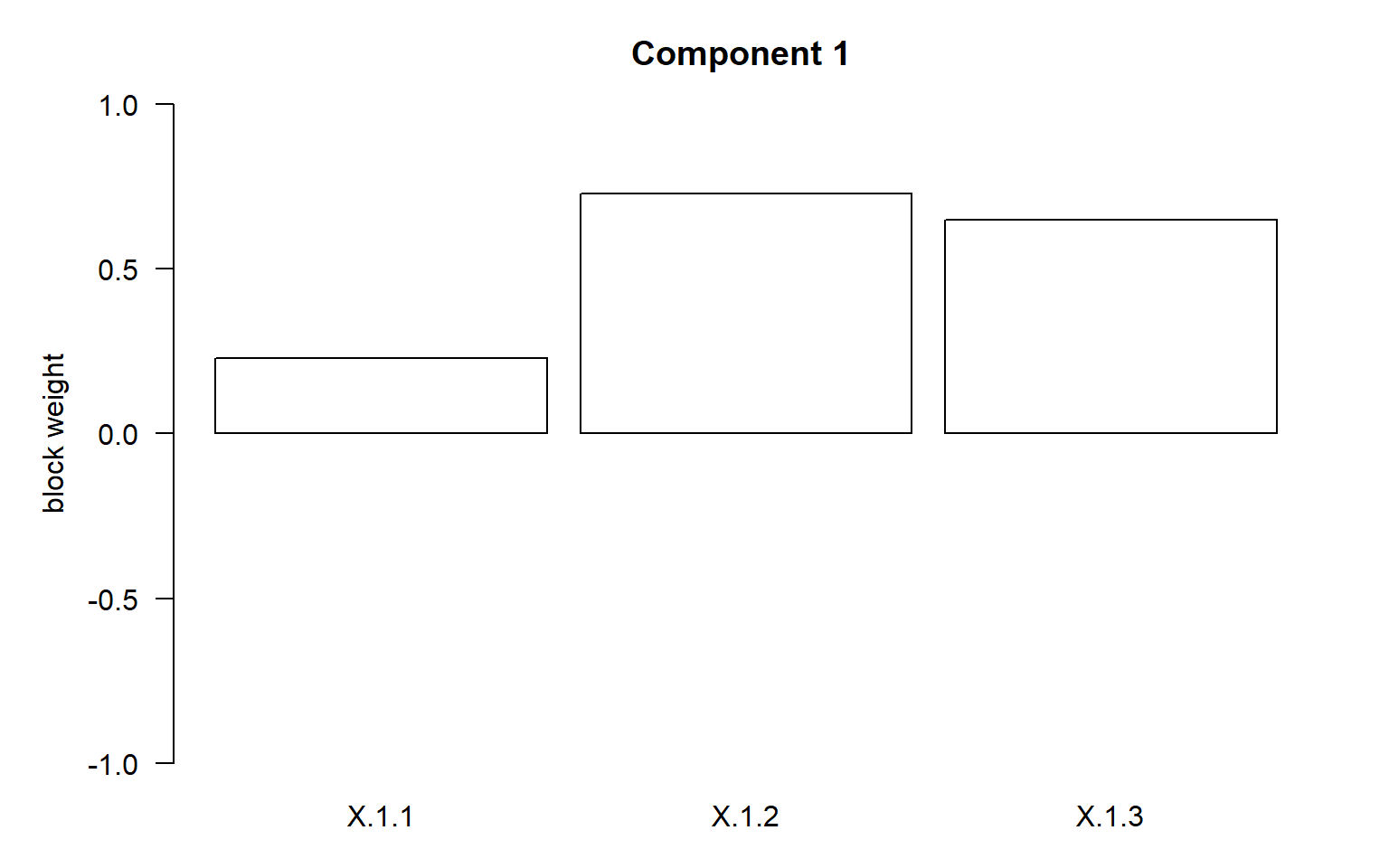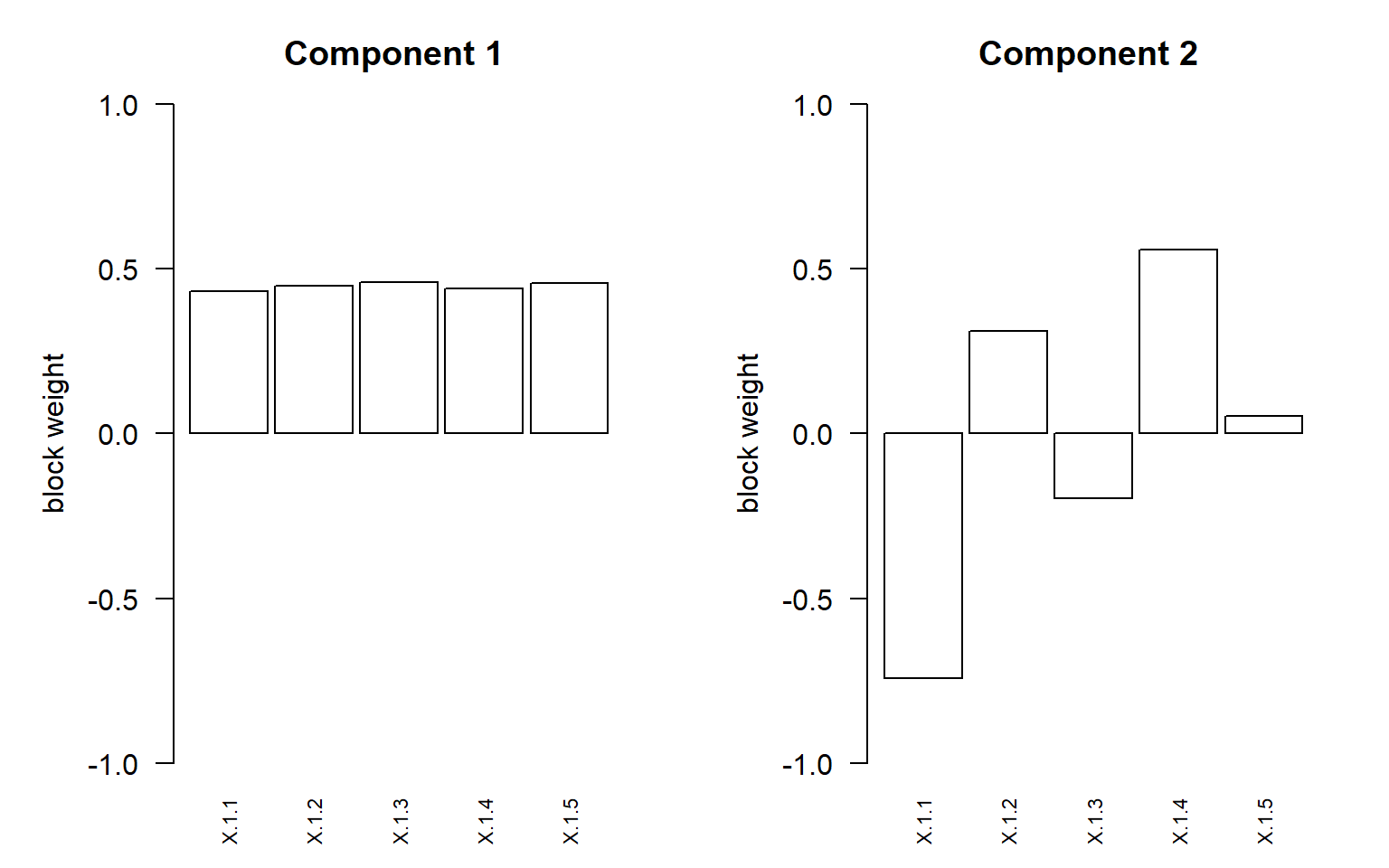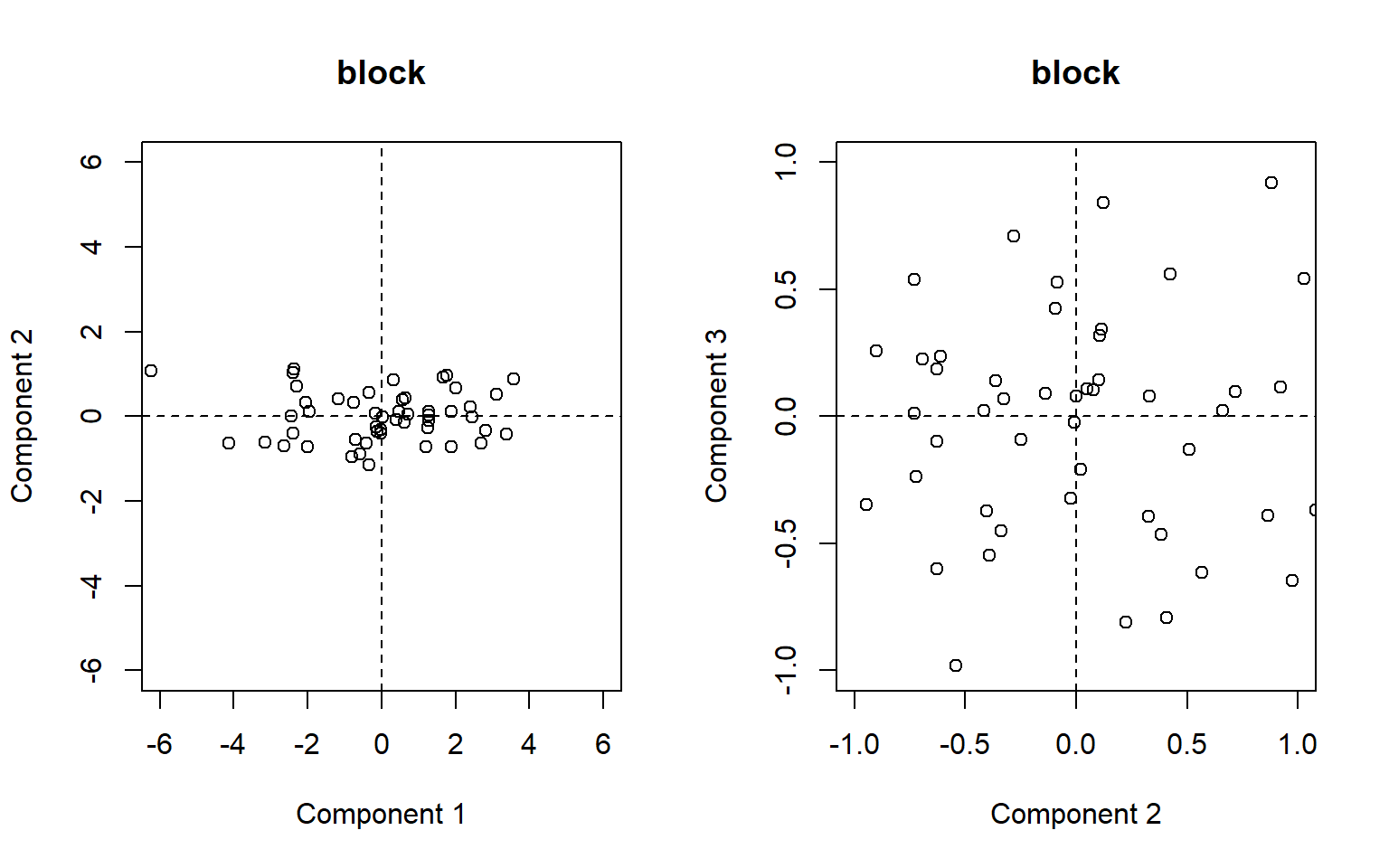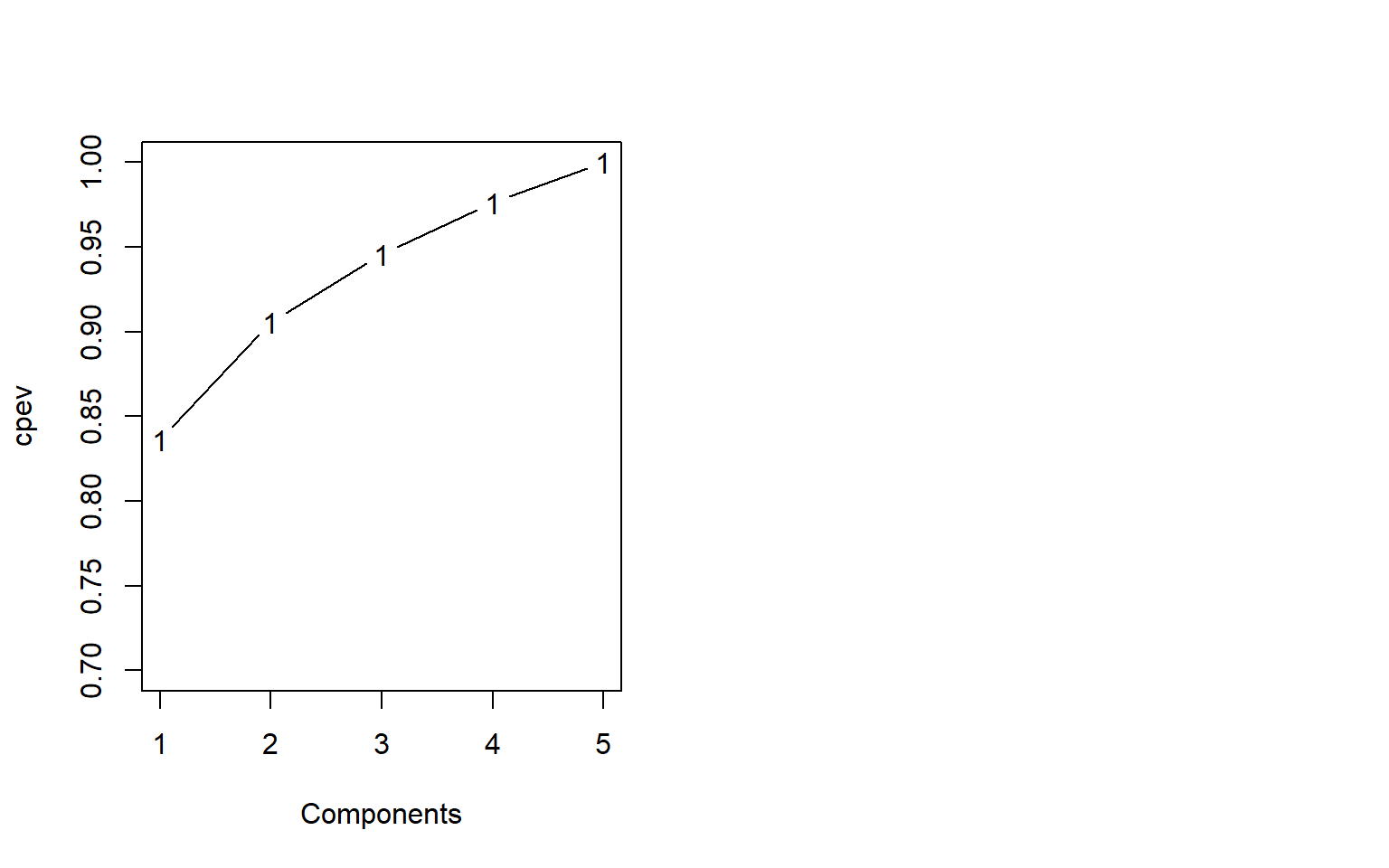2023-08-25 @Atsushi Kawaguchi

The `msma` package provides functions for a matrix decomposition method incorporating sparse and supervised modeling for a multiblock multivariable data analysis.

# Preparation

Install package (as necessary)

``if(!require("msma")) install.packages("msma")``

``library(msma)``

# Getting started

Simulated multiblock data (list data) by using the function `simdata`.

Sample size is 50. The correlation coeficient is 0.8. The numbers of columns for response and predictor can be specified by the argument `Yps` and `Xps`, respectively. The length of vecor represents the number of blocks. That is, response has three blocks with the numbers of columns being 3, 4, and 5 and predictor has one block with the number of columns being 3.

``````dataset0 = simdata(n = 50, rho = 0.8, Yps = c(3, 4, 5), Xps = 3, seed=1)
X0 = dataset0\$X; Y0 = dataset0\$Y ``````

The data generated here is applied to the `msma` function.

## One Component

The argument `comp` can specify the number of components. The arguments `lambdaX` and `lambdaY` can specify the regularization parameters for X and Y, respectively.

First, we set `comp`=1, which will perform an analysis with 1 component.

``````fit01 = msma(X0, Y0, comp=1, lambdaX=0.05, lambdaY=1:3)
fit01``````
``````## Call:
## msma.default(X = X0, Y = Y0, comp = 1, lambdaX = 0.05, lambdaY = 1:3)
##
## Numbers of non-zeros for X block:
##        comp1
## block1     3
##
## Numbers of non-zeros for X super:
##         comp1
## comp1-1     1
##
## Numbers of non-zeros for Y block:
##        comp1
## block1     1
## block2     1
## block3     1
##
## Numbers of non-zeros for Y super:
##         comp1
## comp1-1     3``````

The `plot` function is available. In default setting, the block weights are displayed as a barplot.

``plot(fit01)``## Two Component

Next, we set `comp`=2, which will perform an analysis with 2 components.

``````fit02 = msma(X0, Y0, comp=2, lambdaX=0.03, lambdaY=0.01*(1:3))
fit02``````
``````## Call:
## msma.default(X = X0, Y = Y0, comp = 2, lambdaX = 0.03, lambdaY = 0.01 *
##     (1:3))
##
## Numbers of non-zeros for X block:
##        comp1 comp2
## block1     3     3
##
## Numbers of non-zeros for X super:
##         comp1 comp2
## comp1-1     1     1
##
## Numbers of non-zeros for Y block:
##        comp1 comp2
## block1     3     3
## block2     4     4
## block3     5     5
##
## Numbers of non-zeros for Y super:
##         comp1 comp2
## comp1-1     3     3``````

# Single Block

Two matrics are prepared by specifying arguments `Yps` and `Xps`.

``````dataset1 = simdata(n = 50, rho = 0.8, Yps = 5, Xps = 5, seed=1)
X1 = dataset1\$X[]; Y1 = dataset1\$Y ``````

## Principal Component Analysis (PCA)

If input is a matrix, a principal component analysis is implemented.

``(fit111 = msma(X1, comp=5))``
``````## Call:
## msma.default(X = X1, comp = 5)
##
## Numbers of non-zeros for X block:
##        comp1 comp2 comp3 comp4 comp5
## block1     5     5     5     5     5
##
## Numbers of non-zeros for X super:
##         comp1 comp2 comp3 comp4 comp5
## comp1-1     1     1     1     1     1``````

``fit111\$wbX``
``````## \$block1
##           comp1       comp2       comp3         comp4       comp5
## X.1.1 0.4309622 -0.74170223 -0.03672379  0.1325580413 -0.49520613
## X.1.2 0.4483196  0.31188303  0.63228246  0.5490205405  0.02310504
## X.1.3 0.4601597 -0.19547078 -0.38567734  0.1474129336  0.76129277
## X.1.4 0.4392794  0.55811865 -0.57117598 -0.0006449093 -0.41145448
## X.1.5 0.4566923  0.05386584  0.35196769 -0.8119567864  0.07331836``````

The bar plots of weight vectors are provided by the function `plot`. The component number is specified by the argument `axes`. The plot type is selected by the argument `plottype`. Furthermore, since this function uses the `barplot` function originally built into R, its arguments are also available. In the following example, on the horizontal axis, the magnification of the variable names is set to 0.7 by setting `cex.names`=0.7, and the variable names are oriented as `las`=2.

``````par(mfrow=c(1,2))
plot(fit111, axes = 1, plottype="bar", cex.names=0.7, las=2)
plot(fit111, axes = 2, plottype="bar", cex.names=0.7, las=2)``````The score vectors for first six subjects.

``lapply(fit111\$sbX, head)``
``````## \$block1
##            [,1]          [,2]        [,3]        [,4]        [,5]
## [1,]  0.7097369  0.0487564120  0.10746733 -0.02462727 -0.00598565
## [2,] -0.6976955 -0.5423072581 -0.98211121 -0.23652145 -0.16120137
## [3,]  2.4367362 -0.0238218850 -0.32403419 -0.44206969 -0.47004393
## [4,] -2.4460385 -0.0007036966  0.08112764  0.14263545 -0.45584684
## [5,]  1.7708133  0.9741849574 -0.64716134  0.09377875 -0.78085072
## [6,] -0.8043943 -0.9469205017 -0.34705994 -0.62641753  0.26617649``````

The scatter plots for the score vectors specified by the argument `v`. The argument `axes` is specified by the two length vector represents which components are displayed.

``````par(mfrow=c(1,2))
plot(fit111, v="score", axes = 1:2, plottype="scatter")
plot(fit111, v="score", axes = 2:3, plottype="scatter")``````When the argument `v` was specified as “cpev”, the cummulative eigenvalues are plotted.

``````par(mfrow=c(1,2))
plot(fit111, v="cpev", ylim=c(0.7, 1))``````### Other functions in R for PCA

There is the R function prcomp to implement PCA.

``(fit1112 = prcomp(X1, scale=TRUE))``
``````## Standard deviations (1, .., p=5):
##  2.0446732 0.5899513 0.4458638 0.3926788 0.3439156
##
## Rotation (n x k) = (5 x 5):
##             PC1         PC2         PC3           PC4         PC5
## [1,] -0.4309746  0.74172462 -0.03722419 -1.351296e-01  0.49442882
## [2,] -0.4483141 -0.31171881  0.63044575 -5.510830e-01 -0.02629751
## [3,] -0.4601629  0.19547669 -0.38616901 -1.416349e-01 -0.76213651
## [4,] -0.4392701 -0.55816074 -0.57114566  4.296727e-05  0.41144993
## [5,] -0.4566918 -0.05405032  0.35470924  8.111640e-01 -0.06859643``````
``summary(fit1112)``
``````## Importance of components:
##                           PC1     PC2     PC3     PC4     PC5
## Standard deviation     2.0447 0.58995 0.44586 0.39268 0.34392
## Proportion of Variance 0.8361 0.06961 0.03976 0.03084 0.02366
## Cumulative Proportion  0.8361 0.90575 0.94551 0.97634 1.00000``````

This Rotation is almost the same as the output of `msma`, but it can be made closer by setting the argument `ceps` as follows.

``````fit1113 = msma(X1, comp=5, ceps=0.0000001)
fit1113\$wbX``````
``````## \$block1
##           comp1       comp2       comp3       comp4       comp5
## X.1.1 0.4309745 -0.74172365 -0.03694568  0.13514153 -0.49444798
## X.1.2 0.4483141  0.31172789  0.63155896  0.54980542  0.02621947
## X.1.3 0.4601628 -0.19547781 -0.38588003  0.14252697  0.76211630
## X.1.4 0.4392701  0.55815708 -0.57114810  0.00105051 -0.41145010
## X.1.5 0.4566918  0.05404487  0.35306479 -0.81187175  0.06871165``````

Plotting the scores with the signs turned over, we see that similar scores are calculated.

``````par(mfrow=c(1,2))
biplot(fit1112)
plot(-fit1113\$sbX[][,1:2],xlab="Component 1",ylab="Component 2")``````